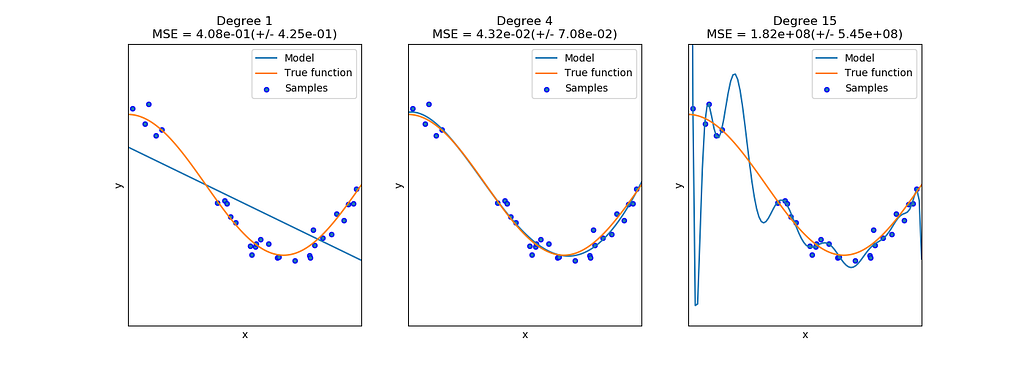# Empirical & Structural Risk Minimization

So you said you want to train a classifier on your data set but you do not know what to optimize on. Let’s say you have the training data set X and its corresponding labels Y, so that you want to train your classifier f that maps incoming data point x (small x means only one data point in the whole set X, similarly for y) to the prediction f(x). You came up with that you can measure the difference between the true label y and the prediction f(x). So naturally you may want to calculate |y-f(x)| — this is absolute loss. The function to measure the loss can be denoted us L. In short, L(Y, f(X))=|Y-f(X)|. There are still many other losses, such as

• 0–1 Loss: L(Y, f(X)) = 1, if Y ≠ f(X) or 0 if Y = f(X),
• Quadratic Loss: L(Y, f(X)) = (Y−f(X))²
• Log-Likelihood Loss: L(Y,P(Y|X)) = −logP(Y|X)

Okay, so now you have picked any one loss listed above, how to use it? Very easy, just calculating the average of the losses of all data points in training set:

Note the h here stands for the f — our classifier. This function is called Empirical Risk Minimization (ERM).

However, if we want to use this classifier to classify the test data (new data points), what if the test data is very different from the training data? Let’s say, we train a object recognizer based on only indoor photos, how can we expect it perform well when showing it a outdoor picture? This is called overfitting — the classifier fit the training data points too perfect to fit the unseen “true” function which we actually want to fit.Fitting the data points by polynomial functions with different degree. The right-most plot shows the classifier fit the data points perfectly while the curve is obviously deviated from the true function which we want to fit. Credit: http://scikit-learn.org/stable/auto_examples/model_selection/plot_underfitting_overfitting.html.

From the plot above it is obvious to see that we can prevent the classifier from overfitting by restricting its complexity. By introducing the regularizer λJ(f) as a penalty term to the equation, the risk function becomes